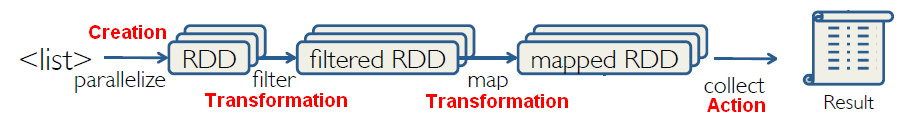# Python - Tuple

Tuples are:

• an ordered sequence of elements, just like lists.
• immutable so they can be elements of sets
• but they can contain mutable objects.

## Constructor

### Basic

A tuple consists of a number of values separated by commas

``````t = 12345, 54321, 'hello!'
t = (1,2,3)  # ordinary parentheses can be used to define a tuple```
```

### Empty

Empty tuples are constructed by an empty pair of parentheses;

``````empty = ()
len(empty)```
```
``````0
```
```

### One item

A tuple with one item is constructed by following a value with a comma (it is not sufficient to enclose a single value in parentheses).

``````singleton = 'hello',    # <-- note trailing comma
len(singleton)```
```
``````1
```
```
````singleton`
```
``````('hello',)
```
```

### From range

With range

``````>>> tuple(range(10))
(0, 1, 2, 3, 4, 5, 6, 7, 8, 9)```
```

### From a list or set

With a list or a set

``````>>> tuple([1,2,3])
(1, 2, 3)
>>> tuple({1,2,3})
(1, 2, 3)```
```

### With a generator

With a generator

``````>>> tuple(2*i for i in range(3))
(0, 2, 4)```
```

## Nested

Tuple may be nested

``````t = (12345, 54321, 'hello!')
u = t, (1, 2, 3, 4, 5) # Tuples may be nested:
u```
```
``````((12345, 54321, 'hello!'), (1, 2, 3, 4, 5))
```
```

## How to

### get value(s) (indexing and slicing)

with indexing and slicing

``````tup = (1, 2, 3, 4, 5, 6, 7 )

print "tup: ", tup # indexing
print "tup[1:5]: ", tup[1:5] # slicing```
```
``````tup:  1
tup[1:5]:  (2, 3, 4, 5)
```
```

### update/delete value(s)

tuples are immutable. You cannot change/delete a value. You have to create a new tuple.

### concat

``````tup1 = (1, 2, 3)
tup2= (4, 5, 6)

print "Concat Tup: ", tup1 + tup2```
```
``````Concat Tup:  (1, 2, 3, 4, 5, 6)
```
```

### Unpacking

``````>>> (a,b) = (1,2)
>>> a
1
>>> b
2```
```

## Comprehension

### Unpacking

``````>>> (y for (x,y) in [(1,'A'),(2,'B'),(3,'C')] )
['A', 'B', 'C']```
```

### Generator

Comprehension cannot be used to create tuple. The below statement is not a comprehension but a generator.

``````>>> (i for i in range(10))
<generator object <genexpr> at 0x02A1F350>```
```

However, a comprehension can be written over a generator instead of over a list or set or tuple.

And the constructor (set(),list(),tuple()) transform a generator into a set or list or tuple.

``````>>> tuple(i for i in range(10))
(0, 1, 2, 3, 4, 5, 6, 7, 8, 9)```
```

### All tuple pairs in a list

``````>>> myList=[1,2,3,4]
>>> {(myList[j], myList[i]) for j in range(len(myList)) for i in range(j,len(myList)) if i != j}
{(1, 2), (1, 3), (1, 4), (2, 3), (3, 4), (2, 4)}```
```

## Documentation / Reference

Discover MorePython - (Dictionary|Map)

A dictionary is similar to a list, but you access values by looking up a key instead of an index. A key can be any string or number. A dictionary is suitable for representing functions with finite domains....Python - (Un)Packing

Initialization and extraction of variables Unpacking: A convenient way to initialize variables from a list Sequence packing: The values 12345, 54321 and 'hello!' are packed together in a tuple...Python - Collection

collection in Python: Data Type: Type Sequence (Ordered) Mutable Duplicate Yes Yes Yes Yes No Yes No Yes No No Yes NoPython - Comprehension (Iteration)

Comprehension are a way to loop over elements of: a , , , tuple, , or . Comprehensions permit to build collections out of other collections. Comprehensions allow one to express computations...Python - List

Lists are used to store a collection of different pieces of information in a sequence order of under a single variable name. A list can be used to implement a . A list is mutable whereas a tuple is not....Python - Sequence (type)

The following data structure are sequence: Sequence objects may be compared to other objects with the same sequence type. The comparison uses lexicographical ordering: first the first two...Python - Set

Implementation of a set data structure in Python. Sets are mutable. There is a non-mutable version of set called frozenset. The elements of a set arenot mutable. A set then cannot contain a list since...Python Type - Dynamic Class

With the three arguments of the type function, you can create dynamically a new class (ie a type object). type is a class that creates class objects...Spark - Key-Value RDD

Spark supports Key-Value pairs RDD in Python trough a list of tuple. A count of an RDD with tuple will return the number of tuples. A tuple can be seen as a row. Some Key-Value Transformations...